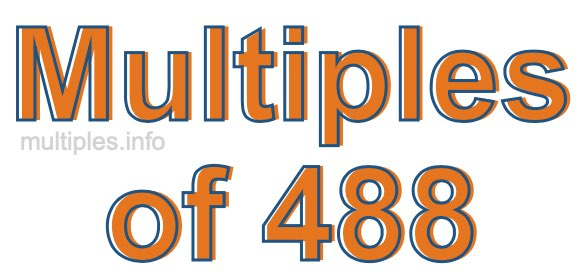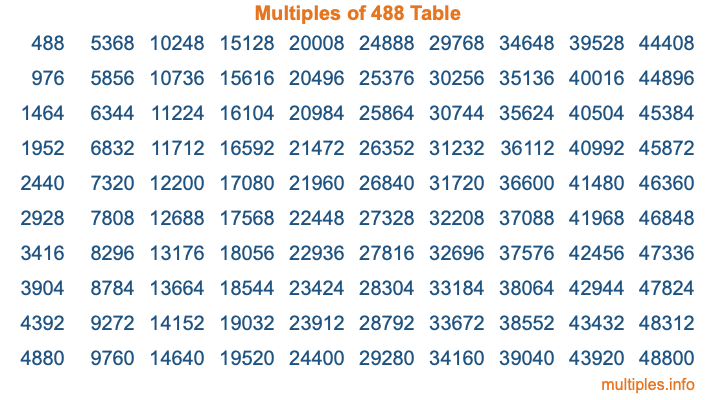Multiples of 488Welcome to the Multiples of 488 page. Here we will first teach you everything you will ever need to know about the multiples of 488, and then give you a study guide summary of everything we taught you to make sure you remember it all. Use this page to look up facts and learn information about the multiples of 488. This page will make you a multiples of four hundred eighty-eight expert!

Definition of Multiples of 488
Multiples of 488 are all the numbers that when divided by 488 equal an integer. Each of the multiples of 488 are called a multiple. A multiple of 488 is created by multiplying 488 by an integer.

Therefore, to create a list of multiples of 488, you start with 1 multiplied by 488, then 2 multiplied by 488, then 3 multiplied by 488, and so on for as long as you want. Thus, the list of the first five multiples of 488 is 488, 976, 1464, 1952, and 2440. To see a larger list of multiples of 488, see the printable image of Multiples of 488 further down on this page. We also have a category where you can choose any nth multiple of 488.

Multiples of 488 Checker
The Multiples of 488 Checker below checks to see if any number of your choice is a multiple of 488. In other words, it checks to see if there is any number (integer) that when multiplied by 488 will equal your number. To do that, we divide your number by 488. If the the quotient is an integer, then your number is a multiple of 488.

Is  a multiple of 488?

Least Common Multiple of 488 and ...
A Least Common Multiple (LCM) is the lowest multiple that two or more numbers have in common. This is also called the smallest common multiple or lowest common multiple and is useful to know when you are adding our subtracting fractions. Enter one or more numbers below (488 is already entered) to find the LCM.

Check out our LCM Calculator if you need more details about the Least Common Multiple or if you need the LCM for different numbers for adding and subtraction fractions.

nth Multiple of 488
As we stated above, 488 is the first multiple of 488, 976 is the second multiple of 488, 1464 is the third multiple of 488, and so on. Enter a number below to find the nth multiple of 488.

th multiple of 488

Multiples of 488 vs Factors of 488
488 is a multiple of 488 and a factor of 488, but that is where the similarities end. All postive multiples of 488 are 488 or greater than 488. All positive factors of 488 are 488 or less than 488.

Below is the beginning list of multiples of 488 and the factors of 488 so you can compare:

Multiples of 488: 488, 976, 1464, 1952, 2440, etc.

Factors of 488: 1, 2, 4, 8, 61, 122, 244, 488

As you can see, the multiples of 488 are all the numbers that you can divide by 488 to get a whole number. The factors of 488, on the other hand, are all the whole numbers that you can multiply by another whole number to get 488.

It's also interesting to note that if a number (x) is a factor of 488, then 488 will also be a multiple of that number (x).

Multiples of 488 vs Divisors of 488
The divisors of 488 are all the integers that 488 can be divided by evenly. Below is a list of the divisors of 488.

Divisors of 488: 1, 2, 4, 8, 61, 122, 244, 488

The interesting thing to note here is that if you take any multiple of 488 and divide it by a divisor of 488, you will see that the quotient is an integer.

Multiples of 488 Table
Below is an image of the first 100 multiples of 488 in a table. The table is in chronological order, column by column. The first column has the first ten multiples of 488, the second column has the next ten multiples of 488, and so on.The Multiples of 488 Table is also referred to as the 488 Times Table or Times Table of 488. You are welcome to print out our table for your studies.

Negative Multiples of 488
Although not often discussed or needed in math, it is worth mentioning that you can make a list of negative multiples of 488 by multiplying 488 by -1, then by -2, then by -3, and so on, to get the following list of negative multiples of 488:

-488, -976, -1464, -1952, -2440, etc.

Multiples of 488 Summary
Below is a summary of important Multiples of 488 facts that we have discussed on this page. To retain the knowledge on this page, we recommend that you read through the summary and explain to yourself or a study partner why they hold true.

There are an infinite number of multiples of 488.

A multiple of 488 divided by 488 will equal a whole number.

488 divided by a factor of 488 equals a divisor of 488.

The nth multiple of 488 is n times 488.

The largest factor of 488 is equal to the first positive multiple of 488.

488 is a multiple of every factor of 488.

488 is a multiple of 488.

A multiple of 488 divided by a divisor of 488 equals an integer.

488 divided by a divisor of 488 equals a factor of 488.

Any integer times 488 will equal a multiple of 488.

Multiples of a Number
Here you can get the multiples of another number, all with the same attention to detail as we did for multiples of 488 on this page.

Multiples of
Multiples of 489
Did you find our page about multiples of four hundred eighty-eight educational? Do you want more knowledge? Check out the multiples of the next number on our list!